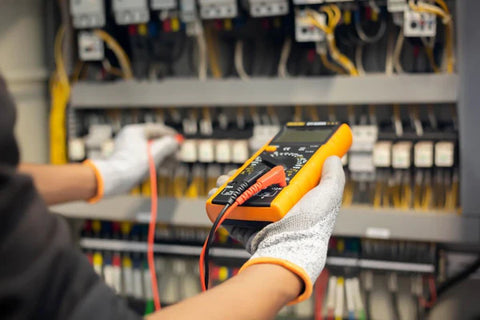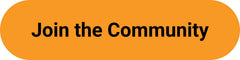Amps To Volt Amps (VA) Calculator - ShopSolar.com

# Amps To Volt Amps (VA) Calculator

Are you new to solar power and installing your own solar system? You’ll come across a few electrical terms that you may not understand, or power rating terminology that you’re unfamiliar with. One of these is the volt amps (VA) rating.

Thankfully, solar power doesn’t have to be complicated. We want to make solar simple and explain how to use an amps to VA calculator, in the guide below. This ensures you only use equipment with the right power ratings for the task at hand.

 Select phase #: Single phase Three phase Enter amps: A Enter line to line volts: V Result in volt-amps: VA

## Amps vs VoltsBefore we proceed, here’s a quick rundown of basic terminology. In electrical circuits, power is measured in volts (V), as well as in amperes (A). It’s important to understand what each of these terms means and how they relate to solar power and electrical circuits.

### Amps

An ampere is the unit of measurement for electric current and describes the number of electrons flowing through a circuit.

When you know the amps, but not the volts, you can convert amps to volts with a simple calculation.

You can also use formulas used in an amp wire gauge calculator to determine the correct wire sizes for solar panel installation.

### VoltsA volt is the unit of measurement for electric potential difference.

It can also be described as the force sending electrons through the circuit to establish the electrical current, which is then measured in amperes.

It’s possible to find all the electrical values you need even when you only have a volts reading with a watts, amps, & volts calculator

## What Are Volt-Amps?

The volt-ampere (VA) rating of a device represents the amount of energy it consumes. In other words, it’s how much current it draws from the electrical circuit.

A volt-ampere (VA) is a measurement of power in a direct current (DC) electrical circuit.  In DC circuits, VA and watts (W) are equal.

The VA measurement is also used in alternating current (AC) circuits. And when it is, it represents apparent power (represented in VA). This apparent power differs from true power (represented in watts).

The power coming out of most solar panels and stored in your solar battery is DC. It gets converted to AC power by an inverter for solar panels. You must always match your inverter's voltage to your battery/ battery bank.

### Why Do VA Ratings Matter?

The volt-ampere (apparent power) is used to simplify power ratings and is often given in the absence of a watt rating. It tells you the power required for electrical devices.

The VA rating should be heeded, as the actual power isn’t always equivalent to the volt-ampere rating. The power supply must have a higher rating than the equipment, to lower the risks of an electrical surge.

## Amps to Volt Amps (VA) Calculator

In any electrical work, there's always a simple formula to get the answers you need. Whether you need to convert amps to watts or convert dc amps to ac amps, we’ve got you covered.

So, now that you understand what voltage amperes are, and why they matter, how do you calculate a VA rating from amps?

• To calculate the VA rating, use the simple amps to VA calculator.
• The apparent power is equal to the voltage times the current in an electrical circuit.
• Apparent Power (VA) = Voltage (V) × Current (A)
• This means that Voltage-Amps = Volts x Amps or put it another way:  VA = V ×A

To calculate voltage amps from amps, you need to do the following:Some solar panels produce AC power, but these panels integrate micro inverters in the panels themselves.

## Conclusion

Once again, you see that solar power can be simple and fun. If you have further questions about installing solar panels or need help choosing the right products for your needs, call us for expert advice.

You can find all of the we have created in order to help you plan, size and install your own solar power system here.

## Join the Solar Lab!